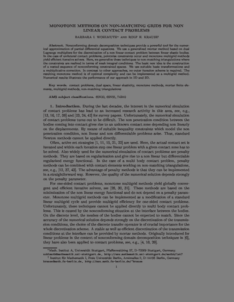Repository: Freie Universität Berlin, Math Department

# Monotone methods on non-matching grids for non-linear contact problems

Wohlmuth, B. and Krause, R. (2003) Monotone methods on non-matching grids for non-linear contact problems. SIAM J. Sci. Comput., 25 (1). pp. 324-347. ISSN 1064-8275Preview

453kB

Official URL: https://dx.doi.org/10.1137/S1064827502405318

## Abstract

Nonconforming domain decomposition techniques provide a powerful tool for the numerical approximation of partial differential equations. We use a generalized mortar method based on dual Lagrange multipliers for the discretization of a nonlinear contact problem between linear elastic bodies. In the case of unilateral contact problems, pointwise constraints occur and monotone multigrid methods yield efficient iterative solvers. Here, we generalize these techniques to nonmatching triangulations, where the constraints are realized in terms of weak integral conditions. The basic new idea is the construction of a nested sequence of nonconforming constrained spaces. We use suitable basis transformations and a multiplicative correction. In contrast to other approaches, no outer iteration scheme is required. The resulting monotone method is of optimal complexity and can be implemented as a multigrid method. Numerical results illustrate the performance of our approach in two and three dimensions.

Item Type: Article Mathematical and Computer Sciences > Mathematics > Numerical Analysis Department of Mathematics and Computer Science > Institute of Mathematics 1836 Ekaterina Engel 15 Apr 2016 12:31 03 Mar 2017 14:42

Repository Staff Only: item control page